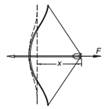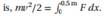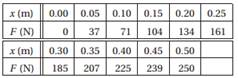### Create an Account

Home / Questions / The table below gives the pull F of the bow as a function of the draw x If the bow is dra...

# The table below gives the pull F of the bow as a function of the draw x If the bow is drawn 05 m determine the speed of the 0075 kg arrow when it leaves the bow Hint the kinetic energy of arrowThe table below gives the pull F of the bow as a function of the draw x. If the bow is drawn 0.5 m, determine the speed of the 0.075-kg arrow when it leaves the bow. Hint: the kinetic energy of arrow equals the work done in drawing the bow; thatJul 27 2020 View more View LessSubscribe To Get Solution